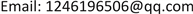1北京建筑大学电气与信息工程学院，北京

2建筑大数据智能处理方法研究北京市重点实验室，北京

3中国科学院软件研究所，北京1. 引言

2. 硬币识别流程设计2.1. 硬币特征分析

2.2. 硬币识别流程

3. 硬币图像预处理3.1. 彩色图像灰度处理

3.2. 图像滤波

4. 边缘检测和图像定位4.1. 边缘检测

G x = [ − 1 0 + 1 − 2 0 + 2 − 1 0 + 1 ] * A (1)

G y = [ − 1 − 2 − 1 0 0 0 + 1 + 2 + 1 ] * A (2)

G = G x 2 + G y 2 (3)

Θ = arctan ( G x G y ) (4)

4.2. 边界定位

r = ( a + b ) / 2 (5)

e = 4π S / C 2 (6)

C = 2 π r (7)

S = π r 2 (8)

5. 硬币识别5.1. 特征提取方法

r / 6 < a < r / 4 (9)

r i = a + d ( i − 1 ) (10)

d = ( r − a ) / 10 (11)

5.2. 存储特征模板

A = { 1 , A > ( A 1 − + A 0 − + A 2 − ) / 3 0 , A ≤ ( A 1 − + A 0 − + A 2 − ) / 3 (12)

135 > ( 123 + 35 + 245 ) / 3 = 134.44 (13)

5.3. 数据库建立

5.4. 特征比对

X = ∑ i = 1 10 ∑ j = 1 3600 | M ( i , j ) − N ( i , j ) | (14)

6. 识别结果及结果分析6.1. 识别结果

6.2. 结果分析

7. 结论